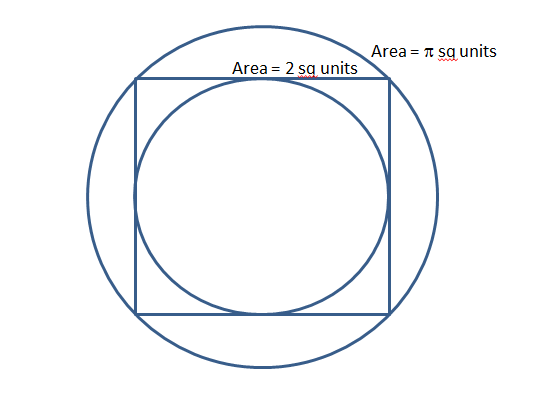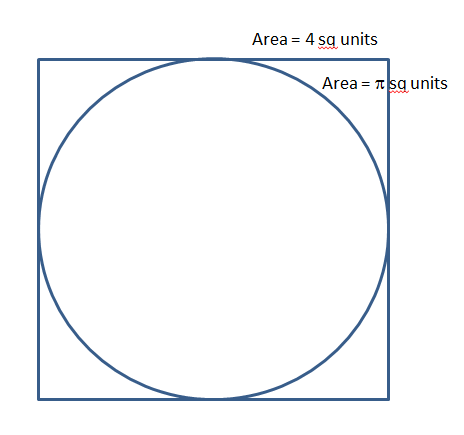# CAT Quantitative Aptitude Questions | Geometry Questions for CAT - Triangles

###### CAT Questions | CAT Geometry Questions | Squares and Circles

The question tests one's knowledge about inscribing a shape inside another. This one focuses, especially on Squares and Circles. CAT Geometry questions are heavily tested in CAT exam. Make sure you master Geometry problems. Triangles are heavily tested, the wonderful infinite-sided polygon that is the circle is also heavily tested. In between these two lies this great mass of regular polygons.

Question 26: Consider Square S inscribed in circle C, what is the ratio of the areas of S and C? And, Consider Circle Q inscribed in Square S, what is the ratio of the areas of S and Q?

1. 2:π, 4:π
2. 4:π, 2:π
3. 1:π, 4:π
4. 2:π, 1:π

## Best CAT Coaching in Chennai

#### CAT Coaching in Chennai - CAT 2021Online Batches Available Now!

##### Method of solving this CAT Question from Regular Hexagon: A very simple Question! Go about it by focussing on the radius of the Circle or the side of the Square.

If square is inside the circle, ratio of areas of square to that of circle is 2 : π.If circle is inside square, ratio of areas of square to that of circle is 4 : π.
Remember, circle area goes with π, square area goes with number.If square is inside the circle, ratio of areas of square to that of circle is 2 : π.
If circle is inside square, ratio of areas of square to that of circle is 4 : π.The question is "What is the ratio of the areas of S and C? and what is the ratio of the areas of S and Q?"

##### Hence, the answer is 2:π, 4:π.

Choice A is the correct answer.

###### CAT Coaching in ChennaiCAT 2021Enroll at 49,000/- 44,000/-

Online Classroom Batches Starting Now!

###### Best CAT Coaching in ChennaiPrices slashed by Rs 4000/-

Attend a Demo Class

## CAT Preparation Online | CAT Geometry questions Videos On YouTube

#### Other useful sources for Geometry Question | Geometry Triangles Circles Quadrilaterals Sample Questions

##### Where is 2IIM located?

2IIM Online CAT Coaching
A Fermat Education Initiative,
58/16, Indira Gandhi Street,
Kaveri Rangan Nagar, Saligramam, Chennai 600 093

##### How to reach 2IIM?

Phone: (91) 44 4505 8484
Mobile: (91) 99626 48484
WhatsApp: WhatsApp Now
Email: prep@2iim.com